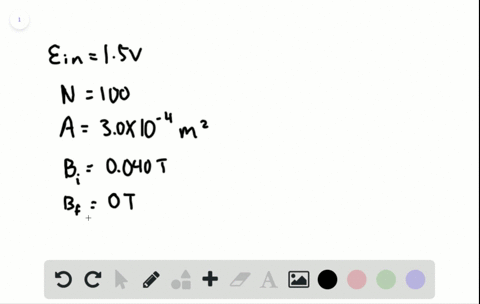🎉 The Study-to-Win Winning Ticket number has been announced! Go to your Tickets dashboard to see if you won! 🎉View Winning Ticket### You want to use the idea of electromagnetic induc…

04:14Indian Institute of Technology Kharagpur
Problem 21

# To measure a magnetic field produced by an electromagnet, you use a circular coil of radius 0.30 $\mathrm{m}$ with 25 loops (resistance of 25$\Omega )$ that rests between the poles of the mag-net and is connected to an ammeter. While the current in the electromagnet is reduced to zero in 1.5 $\mathrm{s}$ , the ammeter in the electromagnet is reduced to zero in 1.5 $\mathrm{s}$ , the ammeter in the coil shows a steady reading of 180 $\mathrm{mA}$ . Draw a picture of the experimental setup and determine everything you can about the electromagnet.

## Discussion

You must be signed in to discuss.

## Video Transcript

In this particulary case, you have some time depend and magnetic field and you have ah, quiet. Over here you have connected to an a meter and he says, Got some resistance. Are the number off turns in the car this known? And the idea off the cornice known the a meter waas found do register current off I So in this particle there circuit electric filled in the circuit is given by I times are the resistance of the oil current ties the resistance of the oil and from the Loops Law Electric field is given by negative changing socks over changing time, which he's negative do de de off number of times in the corner, magnetic field and area. In this case, we're assuming that the oil is head on. Do the manager crude taking the constant terms out we have in a on negative db DT equals I e uh so from here the on the unknown is days so we can figure that out. Dp DT, We don't negative sign is given by I are over and a car and ease 180 million resistance 25 home number of turns 25 and 3.1 forward. Ready a square radius. 0.3 meter, 0.3 squared major square. Okay, so the 25 scans is out. The 25 scans allowed you have God 180 Millie's zero forint. Who on eight. I have got 3.1 fool. Andi, sit upon three square comes out to be 0.9 So all together it becomes 2/3 0.14 week He's Siriporn 637 little born 63 seven. Now, you should understand that this number can be reading as shame in magnetic field over change in time with a negative sign. So negative changing magnetic field be final is zero minus B initial which we want to find over dealt 30 equals b i over. They're 30 on the unit. Over here will be, yes, the over second. So the unknown that we can find from here is B I equals 0.63 seven desa over second Mateu tied by the change in time is given to be 1.5 2nd 1.5 second. So this comes out to be 0.95 five. Yes. No